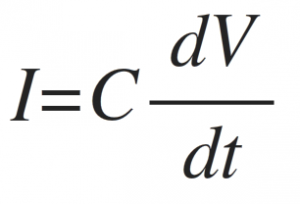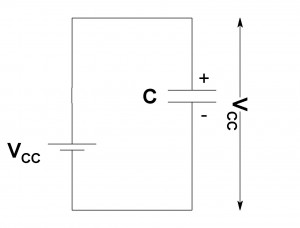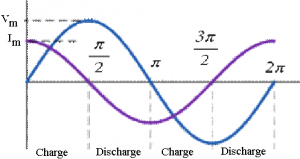Home > electronic components > capacitors > ac analysis of capacitor

# AC Analysis of Capacitor

In capacitor circuit direct current (DC) supply is connected then the capacitor is fully charged up to the DC supply voltage. In vase capacitor store the energy in the form of electric charge. In this circuit the current is flowing through the circuit which is called as charging current due to which the electric charge will be stored at the parallel plates of the capacitor. The charging current is given by,When the capacitor is fully charged due the above charging current then, the capacitor is acts as the DC block device and the charging DC current is blocked in the circuit. Hence, the important property of capacitor is “capacitor blocks the DC supply”. Figure bellow shows the capacitor circuit in which the capacitor is connected with the DC battery source.On the other hand, in a capacitor circuit when alternating current (AC) source is connected, because of positive and negative half cycles of the capacitor, the capacitor will be charged in the positive haf cycle and discharged in the negative half cycle. This charging and discharging of the capacitor depends upon the frequency of the alternating current (AC) source applied in the circuit. From this working of the capacitor it seems that, the capacitance of the capacitor in the AC circuit depends upon the operating frequency of the AC source. Hence, when capacitor is connected in AC circuits and the applied voltage is changing continuously with time the charging current is passed through the capacitor. Therefore another important property of capacitor is “capacitor passes the AC supply”. Figure bellow shows the capacitor circuit in which the capacitor is connected with the AC source.As shown in figure above, the capacitor is connected to the AC supply voltage. When the amplitude of the AC signal increases or decreases the capacitor starts charging and discharging depending upon the frequency. As we have seen that, the charging and discharging current of the capacitor depends upon the rate of change of the supply voltage as can be seen in below equation,When the capacitor the supply voltage crosses from positive cycle to negative cycle or vice versa at points, 0 and 2π along the sine wave, the peak voltage level (Vm ) changes. Hence, the charging current of the circuit also changes as shown below,At 0 angle the AC voltage is increasing in a positive half cycle hence the capacitor charging starts and charging current starts flowing in the circuit. When the AC supply voltage in positive half cycle become Vm at π/2 at this point thre is no any charging and discharging of the capacitor hence no current flows through the capacitor. Beyond π/2 the capacitor starts discharging. At the supply voltage starts decreasing and becomes zero π, the capacitor starts charging in negative direction. Hence this kind of process continues in remaining positive and negative half cycles. Thus, when capacitors are connected in AC circuits the current is zero at maximum value of the supply voltage and the current is maximum when the supply voltage is zero. This clearly shows that, the current in capacitor leads the supply voltage by π/2 or in AC circuits the supply voltage lags the current by π/2.